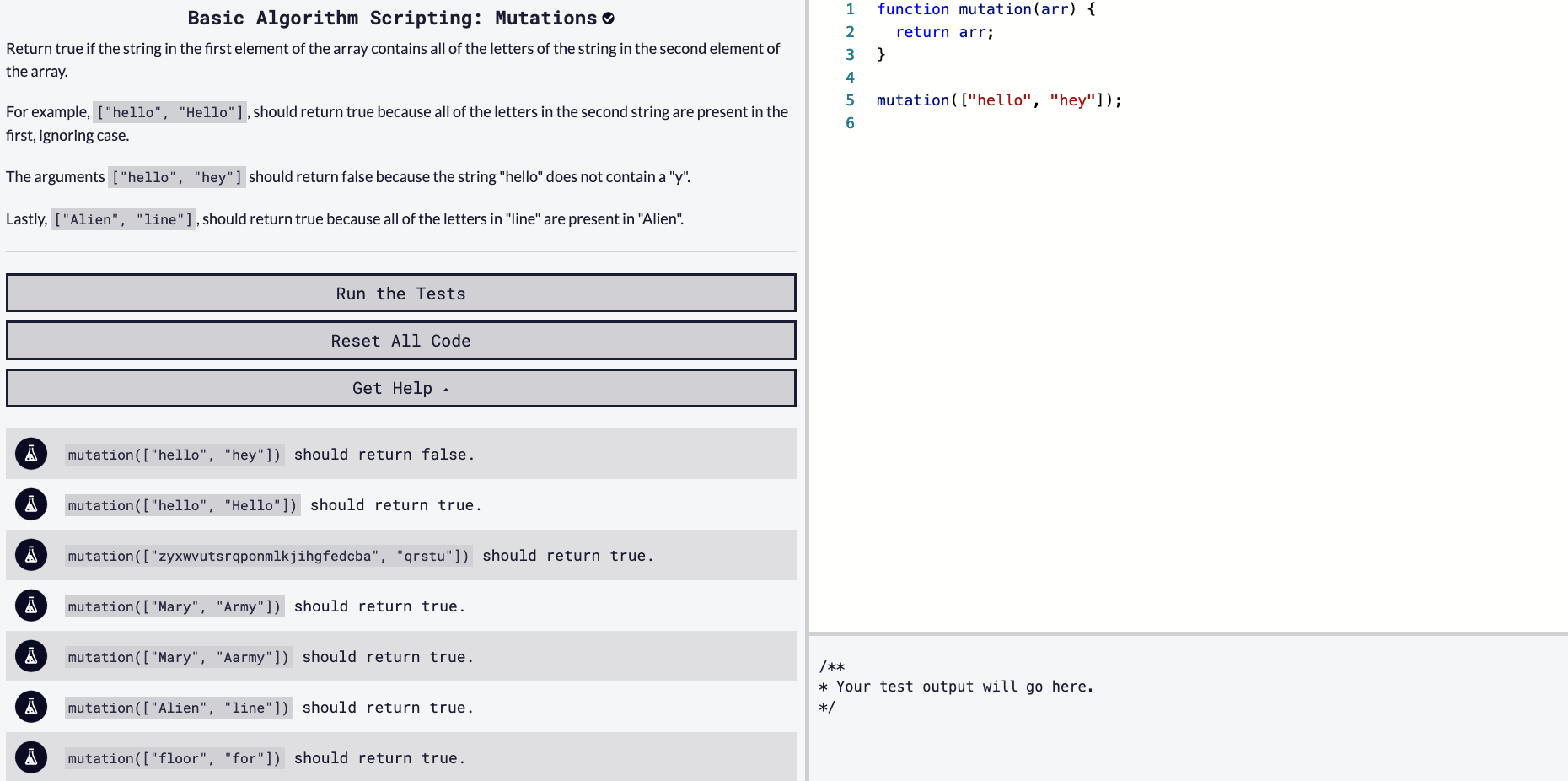## DEV Community is a community of 719,546 amazing developers

We're a place where coders share, stay up-to-date and grow their careers.

Viren B

Posted on • Originally published at virenb.cc

# Solving "Mutations" / freeCodeCamp Algorithm ChallengesLet's solve freeCodeCamp's basic algorithm scripting challenge, 'Mutations'.

### Starter Code

``````function mutation(arr) {
return arr;
}

mutation(["hello", "hey"]);
``````

### Instructions

Return true if the string in the first element of the array contains all of the letters of the string in the second element of the array.

For example, `["hello", "Hello"]`, should return true because all of the letters in the second string are present in the first, ignoring case.

The arguments `["hello", "hey"]` should return false because the string "hello" does not contain a "y".

Lastly, `["Alien", "line"]`, should return true because all of the letters in "line" are present in "Alien".

### Tests

``````mutation(["hello", "hey"]) should return false.
mutation(["hello", "Hello"]) should return true.
mutation(["zyxwvutsrqponmlkjihgfedcba", "qrstu"]) should return true.
mutation(["Mary", "Army"]) should return true.
mutation(["Mary", "Aarmy"]) should return true.
mutation(["Alien", "line"]) should return true.
mutation(["floor", "for"]) should return true.
mutation(["hello", "neo"]) should return false.
mutation(["voodoo", "no"]) should return false.
mutation(["ate", "date"] should return false.
mutation(["Tiger", "Zebra"]) should return false.
mutation(["Noel", "Ole"]) should return true.
``````

# Our Approach

Read everything first. Read the instructions clearly, read the starter code we're given, and read the tests and understand what has to be returned.

• The function takes in one argument, `arr` being an array containing two strings in every case.
• We need to compare the two strings in the array. We need to check if every letter in the second string is in the first string.
• We have to return a boolean value, `true` or `false`.

Now that we understand what we are given and what we want to output, let's see how we can solve this.

How to compare the two values?

The first thing we know, is we can access each string by their index in the array. We should also run `.toLowerCase()` on each string so we are comparing the same characters.

I decided to store each one in new variables.

``````let firstWord = arr.toLowerCase(); // 'hello'
let secondWord = arr.toLowerCase(); // 'hey'
``````

All characters are now lowercased. My next idea is to split `arr` into an array. We will have each character of the string in an index of an array so it would be easier to check if the character is contained in the `arr`.

``````arr.toLowerCase().split(''); // [ "h", "e", "y" ]
``````

We can now loop through the array of characters. I will work with a for loop for this. Within the for loop, we would need to use an if statement to check if the character is in `arr`.

We can use a method, `indexOf()` to check if it is true or false.

Since we want to exit and return false as soon as we find the first character which doesn't exist in the first string, I created a variable called `bool`. It is currently undefined but we will set it to true or false within our if/else statement.

To use `indexOf()`, we can check if the character is contained in the string. If it is not in the string, it will return `-1`.

``````'hey'.indexOf('z') // -1
``````

There is not a 'z' in 'hey' so the above returns `-1`.

MDN Documentation: indexOf()

Here is my mix of pseudo code and some JavaScript:

``````function mutation(arr) {
create empty bool variable
create firstWord variable, equal to arr, lowercased
create secondWord variable, equal to arr, lowercased, split it into an array

for (i = 0; i < secondWord's length; i++) {
if firstWord doesn't contain secondWord[i]
bool = false
return bool (exits the loop)

else
bool = true
}

return bool
}
``````

So to summarize the above:

• Create empty variable to hold our boolean value
• Create variable for `arr` and run `toLowerCase()`
• Create variable for `arr` and run `toLowerCase()`, then run `split('')` to make it into an array
• Create for loop, we want to run it based on secondWord's length
• Within the for loop, create an if statement, using `indexOf()` to check if `arr` character's exist in `arr`
• If it doesn't exist, set `bool` to `false` and return bool
• Set else to `bool = true`
• Return `bool`

# Our Solution [SPOILER: CONTAINS ANSWER]

``````function mutation(arr) {
let bool;
let firstWord = arr.toLowerCase();
let secondWord = arr.toLowerCase().split('');
for (let i = 0; i < secondWord.length; i++) {
if (firstWord.indexOf(secondWord[i]) == -1) {
bool = false;
return bool;
}
else {
bool = true;
}
}
return bool;
}
``````

'Mutations' Challenge on fCC

freeCodeCamp

Donate to FCC!

Solution on my GitHub